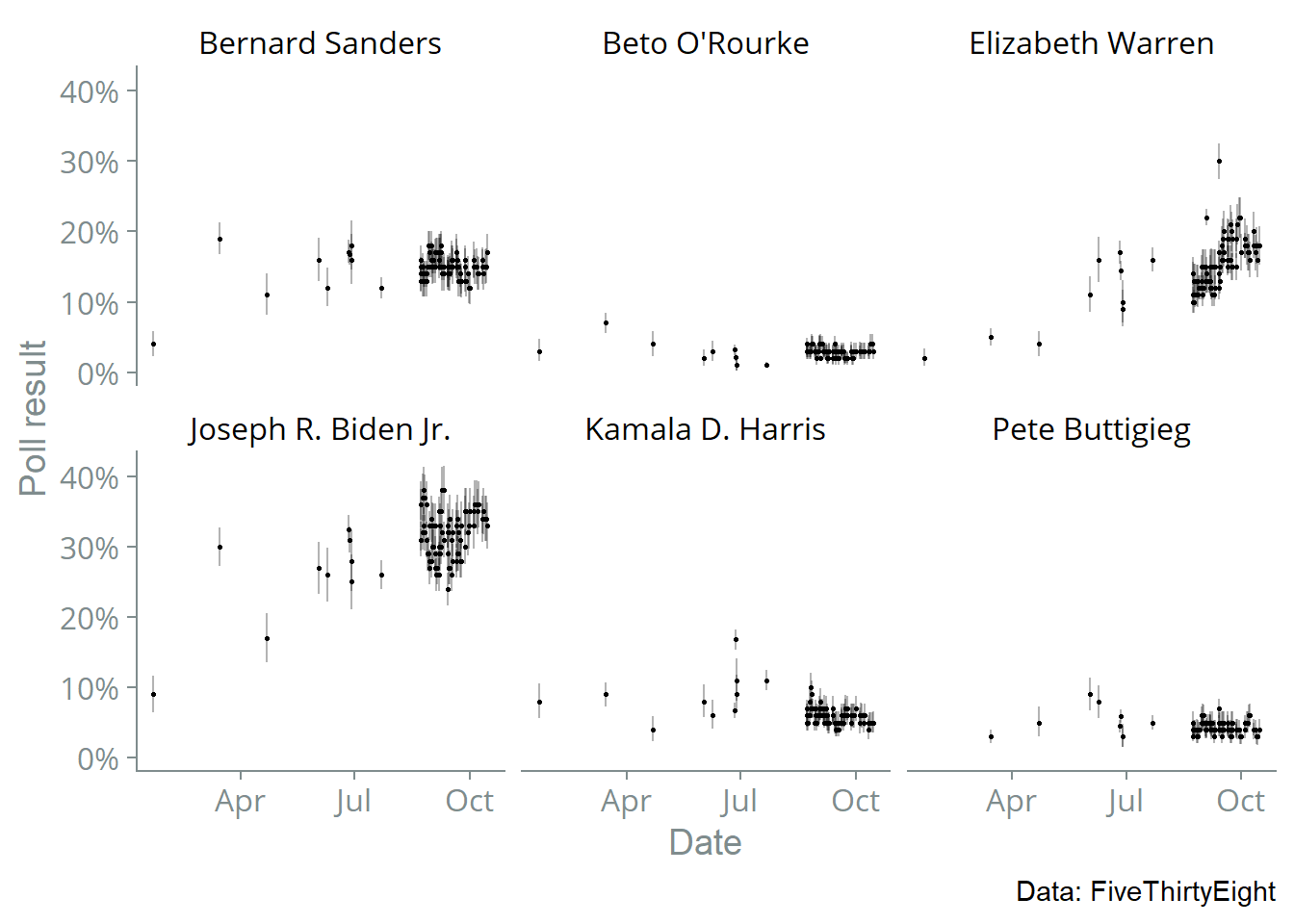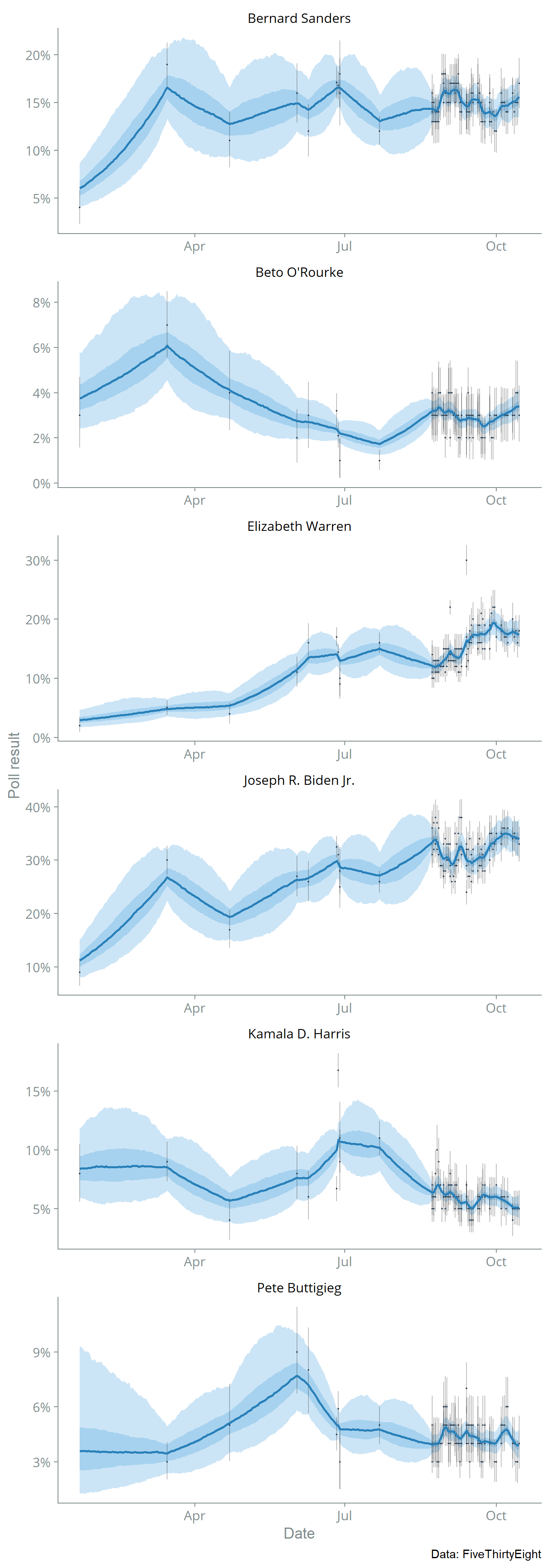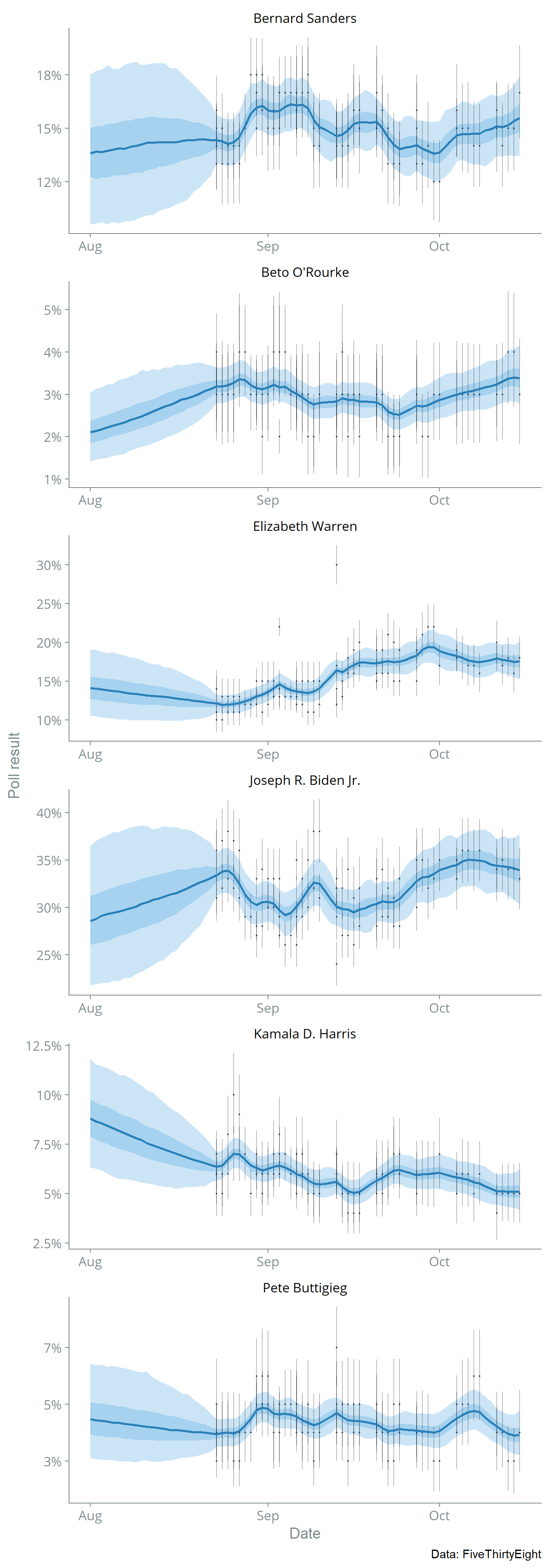# Presidential Primary Polling Analysis in Stan

Note: this post has been updated with more recent data.

I often use random walk/autoregressive models in my research as a component in time-series analysis, and I wanted to get some more experience fitting them to data. FiveThirtyEight publishes several polling datasets, including polling for the 2020 Democratic presidential primary. I used Stan to fit a Bayesian random walk model to the polling data, which I describe below. The Stan and R code used in this post is available as a Github gist.

Let $$\delta_{c,t}$$ be the true proportion of voters in favor of candidate $$c$$ at time $$t$$. Our modeling assumption is that the logit-transform of $$\delta_{c,t}$$ follows a random walk; that is: $\mathrm{logit}(\delta_{c,t}) \sim \mathrm{N}\left(\mathrm{logit}(\delta_{c,t-1}), \tau^2\right)$ We can’t observe $$\delta_{c,t}$$ directly; we have to infer it through the noisy observations we have from polls.

Let $$s_{i}$$ be the sample size of poll $$c[i]$$ and $$y_{i}$$ the number of poll respondents in favor of candidate $$c[i]$$ at time $$t[i]$$. Let $$\phi_i$$ be the proportion of poll respondents in favor of candidate $$c[i]$$ at time $$t[i]$$. To incorporate sampling error, we model $$y_i$$ as binomial: $y_i \sim \mathrm{Binomial}(s_i, \phi_i)$ We also allow for added variance in our observations by relating $$\phi_i$$ to the true logit proportion $$\delta_{c[i], t[i]}$$ with a normal distribution: $\mathrm{logit}(\phi_i) \sim \mathrm{N}(\mathrm{logit}(\delta_{c[i], t[i]}), \sigma^2)$

To finish defining the model half-normal priors on the hyperparameters. The prior for $$\tau^2$$ has a small variance to improve identification of the model (a vaguer prior can cause the MCMC chains to not mix well.) \begin{aligned} \tau^2 &\sim \mathrm{N}(0, 0.02)[0, \infty] \\ \sigma^2 &\sim \mathrm{N}(0, 1)[0, \infty] \end{aligned}

Here is the Stan representation of the statistical model:

S4 class stanmodel 'random_walk' coded as follows:
data {
int<lower=0> T; // Number of timepoints
int<lower=0> C; // Number of candidates
int<lower=0> N; // Number of poll observations

int sample_size[N]; // Sample size of each poll
int y[N]; // Number of respondents in poll for candidate (approximate)
int<lower=1, upper=T> get_t_i[N]; // timepoint for ith observation
int<lower=1, upper=C> get_c_i[N]; // candidate for ith observation
}
parameters {
matrix[C, T] delta_logit; // Percent for candidate c at time t
real<lower=0, upper=1> phi[N]; // Percent of participants in poll for candidate
real<lower=0> tau; // Random walk variance
real<lower=0,upper=0.5> sigma; // Overdispersion of observations
}
model {
// Priors
tau ~ normal(0, 0.2);
sigma ~ normal(0, 1);

// Random walk
for(c in 1:C) {
delta_logit[c, 2:T] ~ normal(delta_logit[c, 1:(T - 1)], tau);
}

// Observed data
y ~ binomial(sample_size, phi);
for(i in 1:N) {
// Overdispersion
delta_logit[get_c_i[i], get_t_i[i]] ~ normal(logit(phi[i]), sigma);
}
}
generated quantities {
matrix[C, T] delta = inv_logit(delta_logit);
} 

The raw dataset that we are going to fit:I fitted the Stan model to the data using the standard HMC-NUTS algorithm and 1,000 MCMC iterations (with 500 of those for warmup.) The plot below shows the posterior median with 75% and 95% credible intervals.Let’s zoom in on August-November 2019 where there are more data:This model is too simple to be that useful, but it could serve as a starting point for more complex models. The model could be improved by incorporating more information, perhaps by finding a data source that includes more polls or modifying the model to incorporate results from head-to-head matchups. We might also want to model systematic biases by the type of poll (phone or internet) or the pollster.